Impact Factor 2.689 | CiteScore 2.27
More on impact ›

# Frontiers in Materials## Original Research ARTICLE

Front. Mater., 09 July 2019 | https://doi.org/10.3389/fmats.2019.00164

# Testing Rigidity Transitions in Glass and Crystal Forming Dense Liquids: Viscoelasticity and Dynamical Gaps

• Departamento de Sistemas Complejos, Instituto de Física, Universidad Nacional Autónoma de México (UNAM), Mexico, Mexico

A computational study of rigidity for dense fluids of monodisperse and bidisperse hard-disks near a phase and a glass transition respectively is presented. To achieve this goal, the transversal part of the dynamical structure factor is calculated. In both cases, a viscoelastic behavior is obtained, with a dynamical gap determined by a critical wavevector kc. Transversal waves exist for k > kc while the maximal correlations happens at frequency ω = 0 for k < kc. In both cases kc goes to zero as the freezing point is approached. Both systems are able to fulfill a scaled dynamical law as a power law is found for the critical kc as a function of the packing. The obtained results indicate that this method gives an alternative to study rigidity and constraint theory in dense fluids, since it is possible to assign a number of floppy modes or broken constraints in the liquid by computing the number of modes below kc, as well as an effective average coordination number. Also, this suggests that the critical wavevector kc can serve as a suitable order parameter.

## 1. Introduction

Over the last years it has become clear that rigidity topology of glass melts is intimately related with its relaxation properties (Selvanathan et al., 2000; Boolchand et al., 2001, 2018; Novita et al., 2007; Rompicharla et al., 2008; Bhosle et al., 2012; Gunasekera et al., 2013; Yildirim et al., 2016), as for example the relationship with fragility, which determines if a compound is good or bad glass former (Mauro et al., 2009, 2014).

Phillips and Thorpe's constraint theory of rigidity gives good insights on how such problems are related to network topology (Phillips, 1979; Thorpe, 1983). These ideas were eventually extended to include thermodynamics (Huerta and Naumis, 2002a,b, 2003; Huerta et al., 2004; Naumis, 2005; Flores-Ruiz and Naumis, 2012; Yan, 2018) and even the Boson peak (Flores-Ruiz and Naumis, 2009, 2011; Flores-Ruiz et al., 2010). Molecular dynamics in realistic systems has been of invaluable help regarding this point (see for instance Bauchy and Micoulaut, 2011). Stochastic models gave similar results (Naumis and Kerner, 1998; Kerner and Naumis, 2000). Boolchand and coworkers have made different studies concerning rigidity in melts and glass aging using optical, mechanical and thermodynamical properties (Selvanathan et al., 2000; Gunasekera et al., 2013).

Rigidity also plays a role in usual thermodynamic phase transitions as any transition involves the development of a generalized rigidity to keep phase order against thermal fluctuations (Chaikin and Lubensky, 1995). In spite of this, even for the usual thermodynamical phase transitions the understanding of the rigidity transition that takes place is not well understood. One of the aims of this paper is precisely this, to study how rigidity evolves for dense fluids near a phase and a glass transition.

Experimentally, rigidity is usually calculated through the average coordination number, obtained from the relative concentration and corresponding valence of each chemical ingredient (Phillips, 1979). Although this is a clear defined protocol in a solid, in a liquid it is more difficult to have such a picture. A simple procedure to surmount such problem is to look not only at the average coordination number, but also to the elastic properties. In fact, the lack of elastic behavior against shear stress turns out to be the main defining feature of a Newtonian fluid. A striking consequence is the absence of transversal waves in fluids at low frequencies and wavenumbers (Trachenko and Brazhkin, 2015; Baggioli et al., 2019). However, elasticity of liquids depends upon the time and spatial scales in which the system is probed or perturbed. Thus, viscoelasticity will contain very valuable information concerning the rigidity of dense liquids close to a glass transition. For organic glasses, there are some early works concerning the study of flexible and rigid polymer models and how this is related with relaxation (Bartenev, 1970; Picu and Weiner, 1998; Picu et al., 1999). For inorganic glasses, this area is still open in many important aspects (Scopigno et al., 2007; Gueguen et al., 2015; Zhou et al., 2017; Zhu et al., 2018; Sen et al., 2019). In this article we will concentrate on how to test rigidity in simple fluids by using the information concerning transversal waves. Such information is encoded in the dynamical structure factor. Here we will compare glass and crystal forming fluids, both in its most elementary aspects.

As previously mentioned, the main feature that defines a rigid system is the resistance to shear-stress. Rigidity relates to the propagation of transversal waves. Thus, a natural starting point is to look at wave propagation, which involves a frequency ω and a wavevector k. Therefore, rigidity of liquids involves time and space density-density and velocity-velocity fluctuations, which are well-described by the dynamical structure factor. Here we are concerned with the transversal part of the dynamical structure factor, defined as (Boon and Yip, 1991),

where CT(k, t) is the transversal current density-density correlation function,

and the brackets 〈…〉 represent an ensemble average. JT(k, t) is the transversal density current averaged over the different directions of k given the wavenumber k = |k|,

Here, vi(t) and ri(t) are the velocity and position of the ith particle of a given system at time t. The $1/\sqrt{2}$ factor takes into account the two transverse currents in three-dimensional systems. This factor is replaced by one in two dimensions.

The resulting correlations functions can also be used to find the complex susceptibility ξ(k, z) (where z = ω + ) when an external field probe F(t) is appplied to the system. It can be proved that the susceptibility is given by (Boon and Yip, 1991),

where β = 1/kBT with T the temperature and kB the Boltzmann constant.

Let us now apply this test to different systems. In particular, here we will start with the most simple fluids able to produce glasses and crystals. One system is made from two dimensional hard-disks and the other is a bidispersive mixture of hard disks. In the monodisperse hard-disks system, the diameter of the disks is σ, while in the bidisperse case there are two kinds of disks, ${A}$ and ${B}$, each with diameters ${\sigma }_{{A}}$ and ${\sigma }_{{B}}$, respectively. For the monodisperse system, the packing fraction is given by η = Nπσ2/A where A is the area. For the bidisperse system $\eta =\left[N\pi \left(x{\sigma }_{{A}}^{2}+\left(1-x\right){\sigma }_{{B}}^{2}\right]/A$, where x is the relative concentration of disks ${A}$. In our simulation, we take x = 1/2 and ${\sigma }_{A}=1.4{\sigma }_{{B}}$. The monodisperse hard-disk system is interesting in many senses as crystallization takes place by two phase transitions. First the liquid goes into coexistence at η ≈ 0.70 with the hexatic phase at η ≈ 0.7175. This is captured by the orientational order parameter, such that in the hexatic phase orientational order appears and the transition is characterized by the Mayer-Wood loop in the Pv diagram (Engel et al., 2013). As the packing fraction increases, a second order transition from hexatic to solid is observed at η ≈ 0.72 (Engel et al., 2013; Russo and Wilding, 2017). While the hexatic phase has short range positional order and quasi-long-range orientational order, the solid has quasi-long range positional order and long-range orientational order. In this regard, positional order parameter is used to distinguish between the hexatic and solid phase (Engel et al., 2013). Recently it has been observed that a small polydispersity destroys the hexatic phase (Russo and Wilding, 2017). Moreover, the bidisperse system at x = 1/2 is able to generate a glass (Isobe, 2016; Russo and Wilding, 2017; Russo et al., 2018). In fact, there is a nice correlation between disk's mismatch, glass forming ability, configurational and vibrational entropy (Russo et al., 2018).

In Figure 1, we present the evolution of the compressibility factor PkBT where ρ = N/A as a function of η. Such results were obtained from an Event-Driven Molecular Dynamics simulation with N = 2, 500 hard-disks. An event driven molecular dynamics simulation called DynamO (Bannerman et al., 2011) was used while, in the case of bidisperse, the initial configuration was generated using the code developed by Skoge et al. (2006). For hard potentials, particles interact only when the distance between them is equal to the sum of their radius. While this condition is not fulfilled, the velocity of the particles remain the same. Event-Driven Molecular Dynamics takes advantage of this by locating the next collision (i.e., the time when the collision will occur and the pair of particles that will collide), evolving the simulation up to that time and implementing the collision dynamics (Allen and Tildesley, 2017). The hydrostatic pressure is computed from the trace of the pressure tensor and divided by 3. However, since we are simulating 2D systems, the element Pzz = 0, therefore, we have rescale by 3/2. The way in which DynamO computes this tensor is from the kinetic and interaction contributions, i.e., the kinetic contribution is defined as

where V is the volume, N the number of disks, mi = 1 is mass, vi is the velocity of disk i and vivi is the dyadic product which yields a matrix. The interaction contribution is defined as

where tsim is the total time of the simulation, the summation is over each two-particle event (collision), i and j indicate the two particles involved in the event, Δpi is the momentum impulse on particle i, and rij = rirj is the separation vector between the interacting particles.

FIGURE 1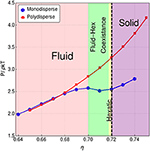Figure 1. Pressure vs. packing fraction in the studied region for monodisperse hard-disks (blue curve) and bidisperse hard-disks (red curve) as shown in the plot legend. For the case of monodisperse hard-disks, below η ≈ 0.7 the system is in the fluid phase (colored pink), between η ≈ 0.7 and η ≈ 0.7175 the fluid coexist with the hexatic phase (colored green). Above η ≈ 0.7175 the system is in a hexatic phase (colored yellow). At η ≈ 0.72, a second order phase transition from hexatic to solid occurs (marked with a dashed line), and above η ≈ 0.72 the system is in the solid phase (colored purple) (Russo and Wilding, 2017). This plot was obtained from a simulation with N = 2, 500 hard-disks.

Figure 1 allows to confirm that, in the case of monodisperse hard-disks, the system is undegoing a first order phase transition, while the bidisperse hard-disk system is not. Our aim is now to look at the viscoelastic response of both systems. In the upper panel of Figure 2, we present the transversal part of the dynamical structure factor S(k, ω) for the monodisperse system near the phase transition. The colors here represent the values of S(k, ω) for different wavenumbers k given in terms of the lowest wavenumber kmin = 2π/L, where $L=\sqrt{\pi \eta /N}4/\sigma$. As k increases, the peaks in S(k, ω) shift to larger values of ω. A gap is seen between the peaks for k ≤ 2kmin. Also, in Figure 2, we compare with the function $\omega \left(k\right)\approx \sqrt{{k}^{2}-{k}_{c}^{2}}$, which shows a good agreement with a recent theoretical solid-state approach to liquids (Trachenko and Brazhkin, 2015; Baggioli and Trachenko, 2018a,b) (see Baggioli et al., 2019 for a recent review on the subject). The lower panel of Figure 2 shows that the bidisperse melt also shows a similar transversal branch with a gap and a critical kc. Recently, this phenomena has been called the dynamical gap (Trachenko and Brazhkin, 2015).

FIGURE 2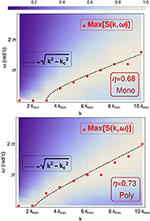Figure 2. Countour plot of the transversal dynamical structure factor S(k, ω) for N = 2, 500 in the case of (Upper panel) monodisperse hard-disk system with packing fraction is η = 0.68 and (Lower panel) polydisperse hard-disk system with packing fraction is η = 0.73. The red dots are the observed maximums. The dotted curve allows to compare with the indicated functional form. Notice the presence of the dynamical gap located at k < 3kmin and k < 2kmin for packing fraction 0.68 monidisperse and packing fraction 0.73 polydisperse, respectively.

Figure 2 gives a nice glimpse of the viscoelasticity and how a transition from a fluid-like to a solid-like behavior is revealed by the presence of a dynamical gap. What is most important to us, is the observation that for k < kc all peaks of S(k, ω) are at ω = 0. Thus, in this region S(k, ω) ≈ δ(ω), where δ(ω) is the Dirac delta function. Since for k < kc we have ω = 0, we can consider these states, in terms of rigidity, as floppy, i.e., the system is flexible. Another way to see this result is by observing that here the rigidity transition will depend upon the time-scale of observation.

To test these ideas, we further follow the behavior of kc as a function of η up to the freezing packing fraction for the monodisperse case. Figure 3 shows the evolution of kc. As expected, kc → 0 as η → ηm, where ηm = 0.7 is the packing fraction where the system becomes solid for this system size. We should stress that for a larger system, kc → 0 at a packing fraction equal to ≈ 0.72 in the case of monodisperse hard-disks, in agreement with the hexatic-to-solid phase transition (Russo and Wilding, 2017). Figure 3 also shows the evolution of kc for the bidisperse case. It is observed that kc → 0 as η → ηp, where ηp = 0.75.

FIGURE 3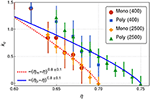Figure 3. Dynamical gap vs. reduced packing fraction η/ηc where ηc es the packing fraction such that kc = kmin. The data points correspond to the simulations while the dashed lines corresponds to fits (see legends). For monodisperse disks ηc = 0.7 while for polydisperse disks ηc = 0.75. The computations were made for systems with N = 400 and N = 2, 500 disks as indicated in the labels.

Thus, Figure 3 clearly shows that a rigidity transition will take place as the fluid density increases. Figure 3 has other interesting features. The first is that the bidisperse fluid presents a bigger dynamical gap for the same given packing fraction. From a rigidity point of views this is expected as the effective number of contacts is reduced. In fact, a previous test in solids showed how one can, by decreasing the size of some disks in a monodisperse system, create a Boson peak (Flores-Ruiz and Naumis, 2009, 2011; Flores-Ruiz et al., 2010). Thus, Figure 3 gives a nice alternative to test in a quantitative way the underlying rigidity of the solid.

Another revealing aspect of Figure 3 is that the critical kc seems to follow the law,

where γ = m or γ = p depending whether the system is monodisperse (m) or polydisperse (p). As kc is the inverse of a dynamical length scale, α represents the scaling of this rigidity, suggesting to be a critical exponent for the size of rigid clusters. Thus, it is expected to depend upon the dimensionality of the system (Toledo-Marín and Naumis, unpublished).

To test this possibility we fitted the curves shown in Figure 3 to the functional from given in Equation (7). We obtained α = 0.8 ± 0.1 in both cases. We, further, fitted kc vs ${\left(\Delta \eta /{\eta }_{\gamma }\right)}^{\alpha }$, where Δη = ηγ − η. In Figure 4, we show the fits and the legend shows the slope values.

FIGURE 4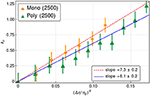Figure 4. Plot of the dynamical gap vs reduced packing fraction ${\left(\Delta \eta /{\eta }_{c}\right)}^{\alpha }$. The data points correspond to the simulations while the dashed lines corresponds to fits (see legends). The computations were made for systems with N = 2, 500 disks.

In the hydrodynamic regime, CL(k, t) Equation (2) satisfies the transverse part of the linearized Navier-Stokes equation. Under very general arguments, it can be proved that the expression for S(k, ω) is given by Boon and Yip (1991),

Here Γ(k) = G(k)/ρ, where G(k) is the wavenumber-dependent high-frequency shear modulus, ρ is the density, ${v}_{0}^{2}={C}_{T}\left(k,t=0\right)$ and τ(k) is the wavenumber-dependent relaxation time (Boon and Yip, 1991).

The condition for shear wave propagation is obtained from equating to zero the derivative of Equation (8) with respect to ω. The resulting inequality for shear wave propagation is,

As k decreases, Γ(k) decreases much faster than τ(k). Thus, the inequality in (9) eventually breaks at a certain kc, such that

In fact, Trachenko and Brazhkin (2015) and Baggioli et al. (2019) studied the dynamical gap and provided a variation to the Navier-Stokes equation, which in turn leads to the well-known telegraph's equation, from that they obtain the following equation for ω,

where τ is a relaxation time. Solving for ω yields

the energy dispersion with a damping and a gap determined by kc = 1/2τVt, a result similar to Equation (10) as ${V}_{t}\approx \sqrt{{G}_{\infty }\left(k\right)/\rho }$ for k >> kc.

Notice that ω has a finite imaginary part. In Figure 2, the frequency corresponds to the real part. Now, from linear response theory, it is easy to see that the inverse of the left-hand-side of Equation (11) is proportional to the susceptibility, hence, by Equation (4) we would expected the imaginary part being encoded in the width of the peaks of the transverse density current correlation function. In Figure 5, we have plotted S(k, ω) vs ω for different values of k, while in Figure 6 we have plotted the width of the transverse density current correlation function vs. the wavenumber, for 2, 500 hard disks with packing fractions η = 0.68 monodisperse and η = 0.73 polydisperse, respectively. As k increases, the width becomes larger, which would imply smaller relaxation times, in agreement with Boon and Yip (1991), Trachenko and Brazhkin (2015), and Baggioli et al. (2019).

FIGURE 5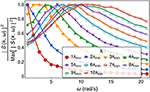Figure 5. The transverse density current correlation function as a function of ω for different wavenumbers. This was obtained for polydisperse hard-disks at a packing fraction 0.73. Notice that for all curves where k < 3kmin, the maximum is located at ω = 0. For k ≥ 3kmin, the peak moves to higher frequencies as k increases, indicating transversal wave propagation although with an increased damping.

FIGURE 6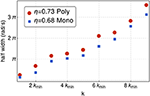Figure 6. Half-width of the transverse density current correlation function as a function of the wavenumber for packing fraction η = 0.68 monodisperse and η = 0.73 polydisperse for 2, 500 hard disks. As k increase, the width becomes larger which is qualitatively consistent with (Trachenko and Brazhkin, 2015; Baggioli et al., 2019).

To test numerically Equation (12) we proceed as follows. Consider for example the case of the polydisperse system for η = 0.68. First Vt is obtained from considering k > > kc from where ω = Vtk. This is the slope of the dotted line in Figure 2, from where Vt ≈ 0.24π/kmin. Next from Figure 6, we obtain that 1/(2τ) ≈ 0.68π. Using Equation (12) we find that kc ≈ 2.83kmin, in good agreement with Figure 2, in which kc ≈ 2kmin.

It is worthwhile to remark that our data satisfy Equation (8), which contains the Maxwell relaxation relationship for viscoelasticity, as it can be proved that in the long wavelength limit, the relaxation time τ(k) is given by Boon and Yip (1991),

where ν is the viscosity. This relation holds for any k dependence of τ(k), even if we make the crude assumption of taking τ(k) = τ(0) = τ.

Let us know return to investigate the connection of constraint theory with the dynamical gap. As the fluid is isotropic, we can obtain a relationship between kc and the number of floppy modes as follows. First we can approximate the behavior for k < kc by a delta function, resulting in a simplified version of S(k, ω),

where Θ(x) is the Heaviside function. The total number of modes with zero frequency in three dimensions is proportional to the volume of a sphere with radius kc in the k-space,

where the factor 2 comes from the possible transversal waves polarizations. In 2D we have ${N}_{f}\left({k}_{c}\right)\approx \pi {k}_{c}^{2}$.

The fraction of floppy modes (f) with respect to the total number of modes is obtained by normalization,

The normalization factor kD(≫kc) is the Debye wavevector (Yang et al., 2017) while the subscripts 3D and 2D refer to the dimensionality of the system. We can conclude that floppy modes are related with the dynamical gap seen in the viscoelastic properties.

Using Equation (7), one can obtain a relationship between η and f valid for the monodisperse and polydisperse system,

In principle, we can go further by relating the previous results to obtain a dynamical average coordination. However, the lattice may have a strong heterogeneous character as floppy regions favor the maximization of vibrational entropy (Naumis, 2005) and care must be taken since it is possible that the system may gain structure and order (Frenkel, 2014) as in the case of the network studied in Yan (2018) where floppy regions appear in a given coordination number window above the rigidity threshold. Relaxation is affected by this heterogeneity (Glasstone et al., 1941; Hänggi et al., 1990; Toledo-Marín and Naumis, 2017, 2018). Yet it is tempting to go further and define a mean dynamical coordination number 〈Z〉. For angular and radial forces are present, is known that f3D = 2−5〈Z〉/6, while f3D = 1−〈Z〉/6 for radial forces (Thorpe, 1983). In 2D, we have for pure radial forces f2D = 1 − 〈Z〉/4. Using Equation (16), for pure radial forces and in 2D we have,

This number can be compared with results obtained from the first-neighbor-counting obtained from collisions (Wyart, 2005). In particular, we observe that for kc = 0 we recover the condition for rigidity, and as kc grows, the coordination number decreases as expected for a fluid system. Furthermore, it is known that the coordination number may be obtained integrating the radial distribution function in a small sphere of radius equal to the distance between two particles. The radial distribution function is related to the structure factor which in turn may be put in terms of the current density correlation function (Boon and Yip, 1991). Thus, it seems plausible to relate the coordination number with the current density correlation function and, in particular, with the dynamical gap. However, we leave that for future work.

In conclusion, here we observed that for simple dense fluids near a glass or a crystal phase transition there is a dynamical gap and above a certain critical wavevector, kc, there are transversal propagating modes although with strong damping. Modes with k below to kc have zero frequency. This critical kc goes to zero as a power law with exponent close to 0.8 as the fluid goes into a solid in the vicinity where this phase transition occurs.

In fact, this sole observation opens new avenues for future research. For example, quite recently it was shown that the hexatic-to-solid phase transition in the case of monodisperse hard-disks, as well as with a small concentration of bidispersity, is a KT transition (Russo and Wilding, 2017). This was proven on the basis of a prediction made within the KTHNY-theory framework, in which the elastic constant, K, should be zero in the hexatic phase and have a jump to 16π in the solid (Strandburg, 1988). This elastic constant K may be expressed in terms of the transverse and longitudinal speed of sound denoted as ${V}_{t}^{2}$ and ${V}_{l}^{2}$, respectively, which yields

When kc goes to zero, shear waves propagate in the system as a whole and the transverse speed of sound changes from zero to some finite value. Hence, in the case of monodisperse hard-disks, we speculate that kc may serve as an order parameter for the hexatic-to-solid transition.

An even more interesting aspect is that, although the hexatic phase disappears for even a small concentration of small hard-disks, the KT transition should still happen theoretically (Strandburg, 1988; Russo and Wilding, 2017). In this sense, the dynamical-gap may serve as a tool to locate it.

Finally, by assuming isotropy of the liquid, one can count zero frequency modes to assign a number of floppy modes to the fluid. Thus, a dynamical average coordination number and a certain number of broken constraints can be defined from this count. Our study suggest that viscoelasticity can serve as a powerful tool to characterize rigidity in the fluid phase.

## Data Availability

The datasets generated for this study are available on request to the corresponding author.

## Author Contributions

GN proposed the work. JT-M did the simulations. JT-M and GN analyzed the results and wrote the manuscript.

## Funding

This work was partially supported by DGAPA-UNAM project IN102717. JT-M acknowledges a doctoral fellowship from CONACyT.

## Conflict of Interest Statement

The authors declare that the research was conducted in the absence of any commercial or financial relationships that could be construed as a potential conflict of interest.

## Acknowledgments

We thank Christian Moukarzel, Le Yan, Adrian Huerta, and John Russo for discussions. We thank the editors for the invitation in order to publish these results.

## References

Allen, M. P., and Tildesley, D. J. (2017). Computer Simulation of Liquids. New York, NY: Oxford University Press.

Baggioli, M., Brazhkin, V., Trachenko, K., and Vasin, M. (2019). Gapped momentum states. arXiv preprint arXiv:1904.01419.

Baggioli, M., and Trachenko, K. (2018a). Maxwell interpolation and close similarities between liquids and holographic models. arXiv preprint arXiv:1808.05391. doi: 10.1103/PhysRevD.99.106002

Baggioli, M., and Trachenko, K. (2018b). Solidity of liquids: how holography knows it. arXiv preprint arXiv:1807.10530.

Bannerman, M. N., Sargant, R., and Lue, L. (2011). Dynamo: a free \calo (n) general event-driven molecular dynamics simulator. J. Comput. Chem. 32, 3329–3338. doi: 10.1002/jcc.21915

Bartenev, G. M. (1970). The Structure and Mechanical Properties of Inorganic Glasses. Transl. by P. Jaray and F. F. Jaray. Groningen: Wolters-Noordhoff Groningen.

Bauchy, M., and Micoulaut, M. (2011). Atomic scale foundation of temperature-dependent bonding constraints in network glasses and liquids. J. Non-Crystall. Solids 357, 2530–2537. doi: 10.1016/j.jnoncrysol.2011.03.017

Bhosle, S., Gunasekera, K., Boolchand, P., and Micoulaut, M. (2012). Melt homogenization and self-organization in chalcogenides-part II. Int. J. Appl. Glass Sci. 3, 205–220. doi: 10.1111/j.2041-1294.2012.00092.x

Boolchand, P., Bauchy, M., Micoulaut, M., and Yildirim, C. (2018). Topological phases of chalcogenide glasses encoded in the melt dynamics (phys. status solidi b 6/2018). Phys. Status Solidi 255:1870122. doi: 10.1002/pssb.201870122

Boolchand, P., Georgiev, D., and Goodman, B. (2001). Discovery of the intermediate phase in chalcogenide glasses. J. Optoelectron. Adv. Mater. 3, 703–720.

Boon, J. P., and Yip, S. (1991). Molecular Hydrodynamics. New York, NY: Courier Corporation.

Chaikin, P. M., and Lubensky, T. C. (1995). Principles of Condensed Matter Physics. Cambridge: Cambridge University Press.

Engel, M., Anderson, J. A., Glotzer, S. C., Isobe, M., Bernard, E. P., and Krauth, W. (2013). Hard-disk equation of state: first-order liquid-hexatic transition in two dimensions with three simulation methods. Phys. Rev. E 87:042134. doi: 10.1103/PhysRevE.87.042134

Flores-Ruiz, H. M., and Naumis, G. G. (2009). Excess of low frequency vibrational modes and glass transition: a molecular dynamics study for soft spheres at constant pressure. J. Chem. Phys. 131:154501. doi: 10.1063/1.3246805

Flores-Ruiz, H. M., and Naumis, G. G. (2011). Boson peak as a consequence of rigidity: a perturbation theory approach. Phys. Rev. B 83:184204. doi: 10.1103/PhysRevB.83.184204

Flores-Ruiz, H. M., and Naumis, G. G. (2012). Mean-square-displacement distribution in crystals and glasses: an analysis of the intrabasin dynamics. Phys. Rev. E 85:041503. doi: 10.1103/PhysRevE.85.041503

Flores-Ruiz, H. M., Naumis, G. G., and Phillips, J. C. (2010). Heating through the glass transition: a rigidity approach to the boson peak. Phys. Rev. B 82:214201. doi: 10.1103/PhysRevB.82.214201

Frenkel, D. (2014). Order through entropy. Nat. Mater. 14:9. doi: 10.1038/nmat4178

Glasstone, S., Eyring, H., and Laidler, K. J. (1941). The Theory of Rate Processes. New York, NY: McGraw-Hill.

Gueguen, Y., Keryvin, V., Rouxel, T., Fur, M. L., Orain, H., Bureau, B., et al. (2015). A relationship between non-exponential stress relaxation and delayed elasticity in the viscoelastic process in amorphous solids: illustration on a chalcogenide glass. Mech. Mater. 85, 47–56. doi: 10.1016/j.mechmat.2015.02.013

Gunasekera, K., Bhosle, S., Boolchand, P., and Micoulaut, M. (2013). Superstrong nature of covalently bonded glass-forming liquids at select compositions. J. Chem. Phys. 139:164511. doi: 10.1063/1.4826463

Hänggi, P., Talkner, P., and Borkovec, M. (1990). Reaction-rate theory: fifty years after kramers. Rev. Modern Phys. 62:251. doi: 10.1103/RevModPhys.62.251

Huerta, A., and Naumis, G. (2002a). Relationship between glass transition and rigidity in a binary associative fluid. Phys. Lett. A 299, 660–665. doi: 10.1016/S0375-9601(02)00519-4

Huerta, A., and Naumis, G. G. (2002b). Evidence of a glass transition induced by rigidity self-organization in a network-forming fluid. Phys. Rev. B 66:184204. doi: 10.1103/PhysRevB.66.184204

Huerta, A., and Naumis, G. G. (2003). Role of rigidity in the fluid-solid transition. Phys. Rev. Lett. 90:145701. doi: 10.1103/PhysRevLett.90.145701

Huerta, A., Naumis, G. G., Wasan, D. T., Henderson, D., and Trokhymchuk, A. (2004). Attraction-driven disorder in a hard-core colloidal monolayer. J. Chem. Phys. 120, 1506–1510. doi: 10.1063/1.1632893

Isobe, M. (2016). Hard sphere simulation in statistical physics-methodologies and applications. Mol. Simul. 42, 1317–1329. doi: 10.1080/08927022.2016.1139106

Kerner, R., and Naumis, G. G. (2000). Stochastic matrix description of the glass transition. J. Phys. 12:1641. doi: 10.1088/0953-8984/12/8/306

Mauro, J. C., Philip, C. S., Vaughn, D. J., and Pambianchi, M. S. (2014). Glass science in the united states: current status and future directions. Int. J. Appl. Glass Sci. 5, 2–15. doi: 10.1111/ijag.12058

Mauro, J. C., Yue, Y., Ellison, A. J., Gupta, P. K., and Allan, D. C. (2009). Viscosity of glass-forming liquids. Proc. Natl. Acad. Sci. U.S.A. 106, 19780–19784. doi: 10.1073/pnas.0911705106

Naumis, G. G. (2005). Energy landscape and rigidity. Phys. Rev. E 71:026114. doi: 10.1103/PhysRevE.71.026114

Naumis, G. G., and Kerner, R. (1998). Stochastic matrix description of glass transition in ternary chalcogenide systems. J. Non-Crystall. Solids 231, 111–119. doi: 10.1016/S0022-3093(98)00417-7

Novita, D. I., Boolchand, P., Malki, M., and Micoulaut, M. (2007). Fast-ion conduction and flexibility of glassy networks. Phys. Rev. Lett. 98:195501. doi: 10.1103/PhysRevLett.98.195501

Phillips, J. C. (1979). Topology of covalent non-crystalline solids I: short-range order in chalcogenide alloys. J. Non-Crystall. Solids 34, 153–181. doi: 10.1016/0022-3093(79)90033-4

Picu, R. C., Loriot, G., and Weiner, J. H. (1999). Toward a unified view of stress in small-molecular and in macromolecular liquids. J. Chem. Phys. 110, 4678–4686. doi: 10.1063/1.478351

Picu, R. C., and Weiner, J. H. (1998). Stress relaxation in a diatomic liquid. J. Chem. Phys. 108, 4984–4991. doi: 10.1063/1.475907

Rompicharla, K., Novita, D., Chen, P., Boolchand, P., Micoulaut, M., and Huff, W. (2008). Abrupt boundaries of intermediate phases and space filling in oxide glasses. J. Phys. 20:202101. doi: 10.1088/0953-8984/20/20/202101

Russo, J., Romano, F., and Tanaka, H. (2018). Glass forming ability in systems with competing orderings. Phys. Rev. X 8:021040. doi: 10.1103/PhysRevX.8.021040

Russo, J., and Wilding, N. B. (2017). Disappearance of the hexatic phase in a binary mixture of hard disks. Phys. Rev. Lett. 119:115702. doi: 10.1103/PhysRevLett.119.115702

Scopigno, T., Yannopoulos, S. N., Scarponi, F., Andrikopoulos, K. S., Fioretto, D., and Ruocco, G. (2007). Origin of the λ transition in liquid sulfur. Phys. Rev. Lett. 99:025701. doi: 10.1103/PhysRevLett.99.025701

Selvanathan, D., Bresser, W., and Boolchand, P. (2000). Stiffness transitions in si x se 1- x glasses from raman scattering and temperature-modulated differential scanning calorimetry. Phys. Rev. B 61:15061. doi: 10.1103/PhysRevB.61.15061

Sen, S., Xia, Y., Zhu, W., Lockhart, M., and Aitken, B. (2019). Nature of the floppy-to-rigid transition in chalcogenide glass-forming liquids. J. Chem. Phys. 150:144509. doi: 10.1063/1.5092841

Skoge, M., Donev, A., Stillinger, F. H., and Torquato, S. (2006). Packing hyperspheres in high-dimensional euclidean spaces. Phys. Rev. E 74:041127. doi: 10.1103/PhysRevE.74.041127

Strandburg, K. J. (1988). Two-dimensional melting. Rev. Modern Phys. 60:161. doi: 10.1103/RevModPhys.60.161

Thorpe, M. (1983). Continuous deformations in random networks. J. Non-Crystall. Solids 57, 355–370. doi: 10.1016/0022-3093(83)90424-6

Toledo-Marín, J. Q., and Naumis, G. G. (2017). Short time dynamics determine glass forming ability in a glass transition two-level model: a stochastic approach using kramers– escape formula. J. Chem. Phys. 146:094506. doi: 10.1063/1.4977517

Toledo-Marín, J. Q., and Naumis, G. G. (2018). Escape time, relaxation, and sticky states of a softened henon-heiles model: Low-frequency vibrational mode effects and glass relaxation. Phys. Rev. E 97:042106. doi: 10.1103/PhysRevE.97.042106

Trachenko, K., and Brazhkin, V. (2015). Collective modes and thermodynamics of the liquid state. Rep. Progr. Phys. 79:016502. doi: 10.1088/0034-4885/79/1/016502

Wyart, M. (2005). On the Rigidity of Amorphous Solids. Price Fluctuations, Conventions and Microstructure of Financial Markets. Physics and Society [physics.soc-ph]. Ecole Polytechnique X, ffpastel-00001919. Available online at: https://pastel.archives-ouvertes.fr/pastel-00001919/document

Yan, L. (2018). Entropy favors heterogeneous structures of networks near the rigidity threshold. Nat. Commun. 9:1359. doi: 10.1038/s41467-018-03859-9

Yang, C., Dove, M., Brazhkin, V., and Trachenko, K. (2017). Emergence and evolution of the k gap in spectra of liquid and supercritical states. Phys. Rev. Lett. 118:215502. doi: 10.1103/PhysRevLett.118.215502

Yildirim, C., Micoulaut, M., Boolchand, P., Kantor, I., Mathon, O., Gaspard, J.-P., et al. (2016). Universal amorphous-amorphous transition in ge x se 100- x glasses under pressure. Sci. Rep. 6:27317. doi: 10.1038/srep27317

Zhou, T., Zhou, Q., Xie, J., Liu, X., Wang, X., and Ruan, H. (2017). Elastic-viscoplasticity modeling of the thermo-mechanical behavior of chalcogenide glass for aspheric lens molding. Int. J. Appl. Glass Sci. 9, 252–262. doi: 10.1111/ijag.12290

Zhu, W., Aitken, B. G., and Sen, S. (2018). Communication: observation of ultra-slow relaxation in supercooled selenium and related glass-forming liquids. J. Chem. Phys. 148:111101. doi: 10.1063/1.5022787

Keywords: dynamical-gap, rigidity, relaxation, hard-disks, viscoelasticity

Citation: Toledo-Marín JQ and Naumis GG (2019) Testing Rigidity Transitions in Glass and Crystal Forming Dense Liquids: Viscoelasticity and Dynamical Gaps. Front. Mater. 6:164. doi: 10.3389/fmats.2019.00164

Received: 14 May 2019; Accepted: 24 June 2019;
Published: 09 July 2019.

Edited by:

Punit Boolchand, University of Cincinnati, United States

Reviewed by:

Mathieu Bauchy, University of California, Los Angeles, United States
Matteo Baggioli, Institute of Theoretical Physics, Faculty of Science, Autonomous University of Madrid, Spain

Copyright © 2019 Toledo-Marín and Naumis. This is an open-access article distributed under the terms of the Creative Commons Attribution License (CC BY). The use, distribution or reproduction in other forums is permitted, provided the original author(s) and the copyright owner(s) are credited and that the original publication in this journal is cited, in accordance with accepted academic practice. No use, distribution or reproduction is permitted which does not comply with these terms.

*Correspondence: Gerardo G. Naumis, naumis@fisica.unam.mx